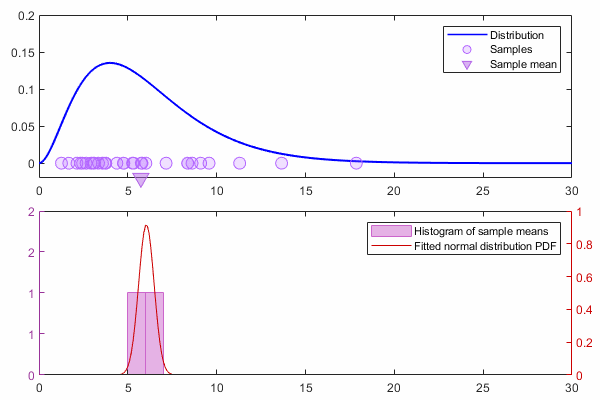## Probability-Theory

version 1.1.3 (494 KB) by
A courseware module that covers fundamental concepts including the basics of probability, random variables, and Bayes' Theorem.

Updated 31 Jan 2022

From GitHub

# Fundamentals of Probability TheoryCurriculum Module
Created with R2021a. Compatible with R2021a and later releases.

## Description

This package contains live scripts and supporting files to teach the fundamental concepts in probability theory and statistical inference. These materials are designed to be flexible and can be easily modified to accommodate a variety of teaching and learning methods. Used in a sequence, the live scripts progressively add depth to the topic. However, each script or section can be easily adapted for standalone use. The live scripts include a brief background, interactive illustrations, tasks, reflection questions, and application examples.

This module can be used as part of a lecture, as activities in an instructional setting, or as interactive assignments to be completed outside of class. The live script sections contains direct instructions or tasks to illustrate the basics and additional reflection questions that are more open-ended.

Get started with each live script by running it one section at a time. To stop running the script or a section midway (for example, when an animation is in progress), use the Stop button in the Run section of the Live Editor tab in the MATLAB toolstrip.

## Prerequisite Domain Knowledge

This module assumes a knowledge of basic concepts in set theory, such as ⋃ (union) and ⋂ (intersection). It also assumes basic fluency in mathematical notation for Σ (summation) and Π (product). Other notation relevant to probability theory is introduced within the module as needed.

## Suggested Prework

MATLAB Onramp – a free two-hour introductory tutorial to learn the essentials of MATLAB®.

## Details

`probabilityIntro.mlx`
Learning Goals

`randomVariables.mlx`, `randomVariableApp.mlapp`
Learning Goals

• Define and create random variables to describe random processes.
• Generate an empirical distribution of random variables through random sampling.
• Explain the meaning of expectation and variance for random variables.
• Explain the difference between continuous and discrete random variables.
• Identify and use the discrete uniform and continuous normal probability distributions.
• Define and apply the probability density function and cumulative density function to find the probability of a random variable's value falling within a given interval.
• State the Central Limit Theorem and explain its implications for statistical inference.`inferenceBayes.mlx`
Learning Goals

Data files: `people.png`(optional)

## Products

MATLAB, Statistics and Machine Learning Toolbox™, Image Processing Toolbox™

The license for this module is available in the LICENSE.TXT file in this GitHub repository.

## Educator Resources

Have any questions or feedback? Contact the MathWorks online teaching team.

Authored by: Pooja Lalan
Maintained by: Emma Smith Zbarsky

### Cite As

Emma Smith Zbarsky (2022). Probability-Theory (https://github.com/MathWorks-Teaching-Resources/Probability-Theory/releases/tag/v1.1.3), GitHub. Retrieved .

##### MATLAB Release Compatibility
Created with R2021a
Compatible with any release
##### Platform Compatibility
Windows macOS Linux